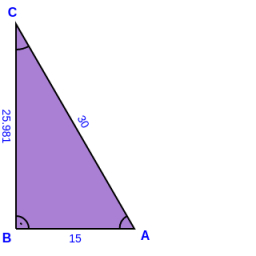# Hypotenuse 64694

Point S is the center of the hypotenuse AB of the right triangle ABC. Calculate the content of triangle ABC if the line on the hypotenuse is 0.2 dm long and if | ∢ACS | = 30 °.

S =  3.4641 cm2

### Step-by-step explanation:

Try calculation via our triangle calculator.Did you find an error or inaccuracy? Feel free to write us. Thank you!

Tips for related online calculators
Need help calculating sum, simplifying, or multiplying fractions? Try our fraction calculator.
Do you know the volume and unit volume, and want to convert volume units?
Do you want to convert time units like minutes to seconds?